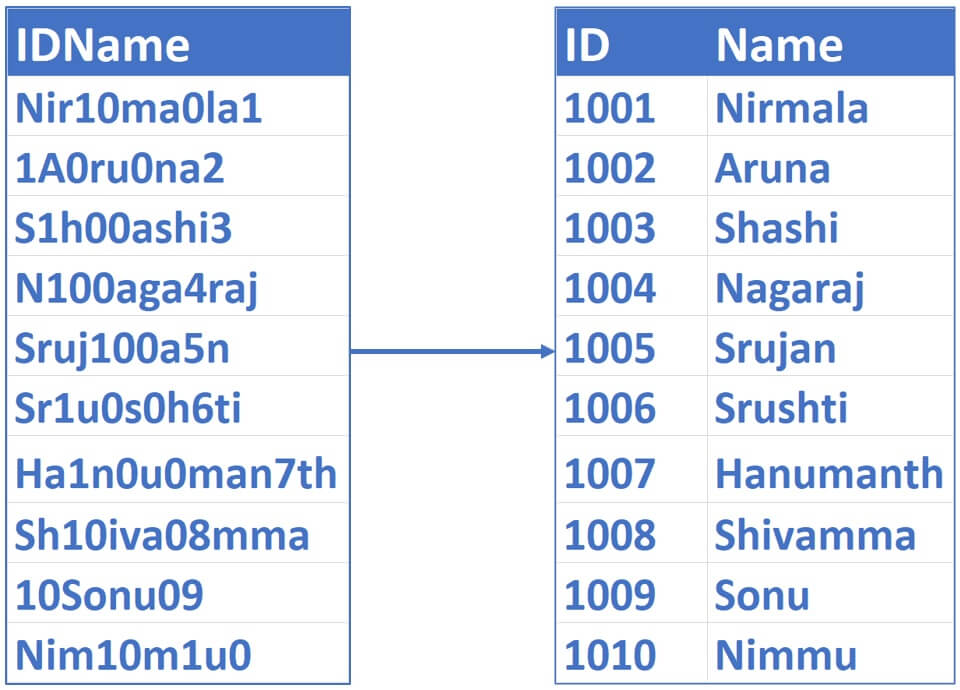# Sql function to get number from string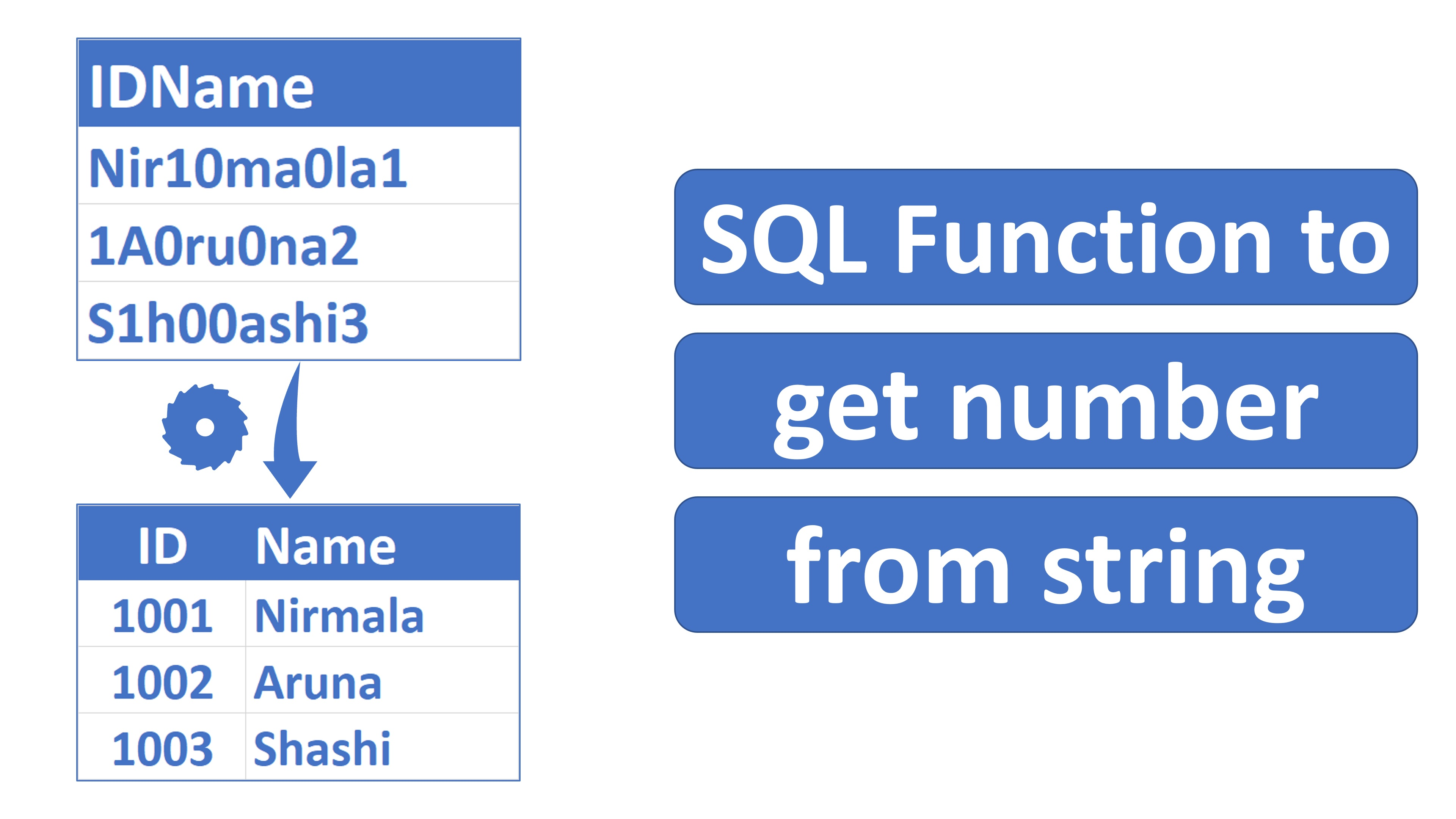In this video we will discuss two common tasks that are usually asked in a SQL interview.

1. Write a sql query to extract only alphabets from a given alphanumeric string.
2. Write a sql query to extract only numbers from a given alphanumeric string.

This table has only one column and it contains both numbers and alphabets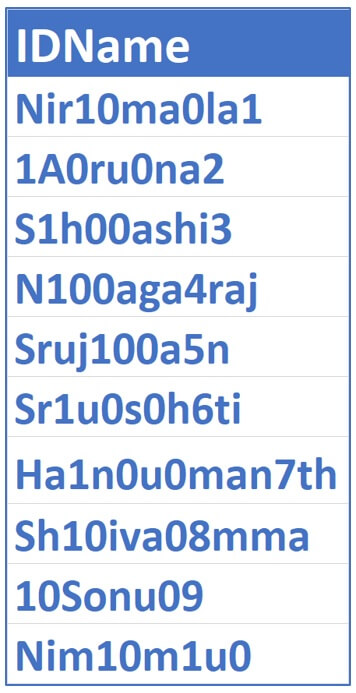Now, the task at hand is to write a query that  extracts numbers and letters into separate columns as you can see below.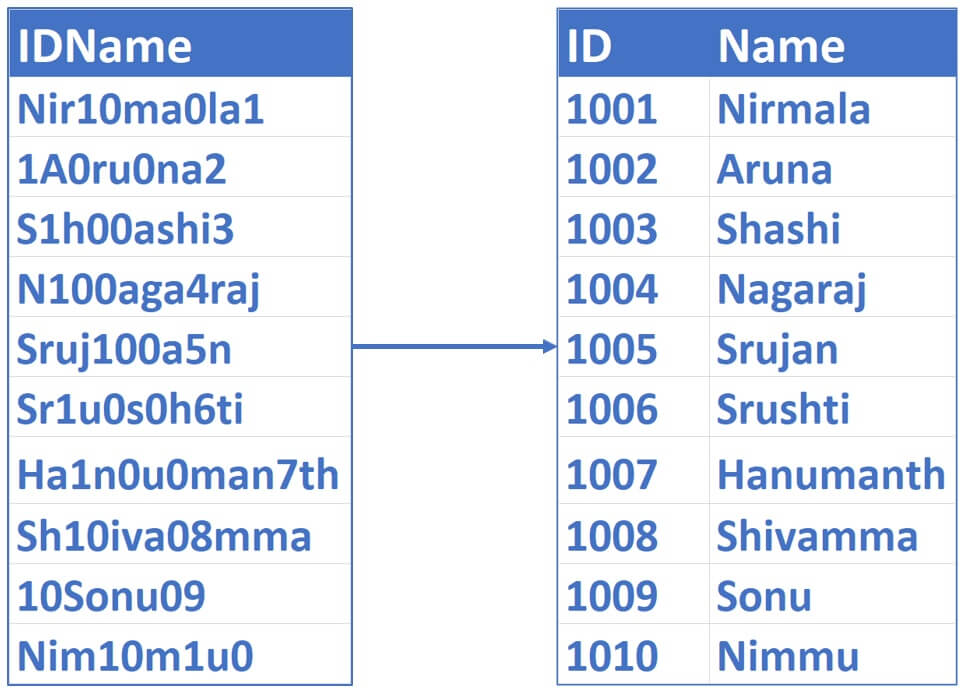If you want to follow along, the following is the SQL script to create the table and populate it with test data.

``````Create table TestTable
(
IDName nvarchar(25)
)
Go

Insert into TestTable values('Nir10ma0la1')
Insert into TestTable values('1A0ru0na2')
Insert into TestTable values('S1h00ashi3')
Insert into TestTable values('N100aga4raj')
Insert into TestTable values('Sruj100a5n')
Insert into TestTable values('Sr1u0s0h6ti')
Insert into TestTable values('Ha1n0u0man7th')
Insert into TestTable values('Sh10iva08mma')
Insert into TestTable values('10Sonu09')
Insert into TestTable values('Nim10m1u0')
Go``````

With this SQL interview question, the interviewer is basically checking if you know the following concepts

• User Defined Functions and
• How to use SQL Server built-in functions - PatIndex() and Stuff()We discussed User Defined Functions in detail in our SQL Server Tutorial for beginners course. Please check videos from Parts 30 to 33. We also discussed PatIndex() and Stuff() functions in Part 24. The following is the link to SQL Server course page.

SQL Server tutorial for beginners

## Patindex in SQL

• PATINDEX('%Pattern%', Expression)
• Returns the starting position of the first occurrence of a pattern in a specified expression. It takes two arguments, the pattern to be searched and the expression.

## Stuff in sql server

• STUFF(Original_Expression, Start, Length, Replacement_expression)
• STUFF() function inserts Replacement_expression, at the start position specified, along with removing the charactes specified using Length parameter.

## SQL function to extract numbers from an alphanumeric string

``````Create function UDF_ExtractNumbers
(
@input varchar(255)
)
Returns varchar(255)
As
Begin
Declare @alphabetIndex int = Patindex('%[^0-9]%', @input)
Begin
While @alphabetIndex > 0
Begin
Set @input = Stuff(@input, @alphabetIndex, 1, '' )
Set @alphabetIndex = Patindex('%[^0-9]%', @input )
End
End
Return @input
End``````

## Same function with code explanation

``````Create function UDF_ExtractNumbers
(
-- Input is alphanumeric string
@input varchar(255)
)
-- Returns numbers as a string
Returns varchar(255)
As
Begin
-- Returns the index of a character that is not a number
Declare @alphabetIndex int = Patindex('%[^0-9]%', @input)
Begin
While @alphabetIndex > 0
Begin
-- In the input string (@input) at the position (@alphabetIndex)
-- where we have a non-numeric chracter, replace that 1
-- character with an empty string ('')
Set @input = Stuff(@input, @alphabetIndex, 1, '' )
-- Find the next non-numeric character and repeat the above step
-- until all non-numeric characters are replaced with an empty string
Set @alphabetIndex = Patindex('%[^0-9]%', @input )
End
End
Return @input
End``````

## Using the user defined function in a query

``Select dbo.UDF_ExtractNumbers(IDName) as ID as Numbers from TestTable``

## Result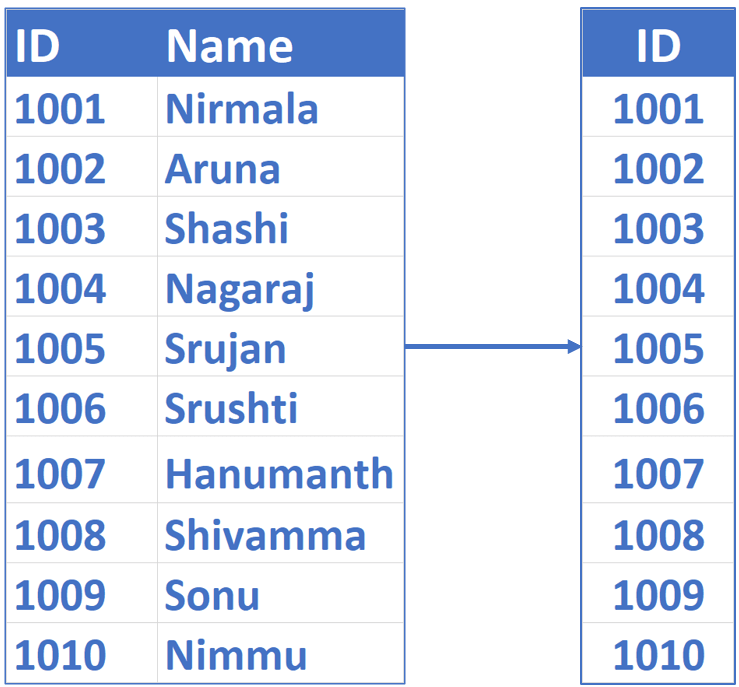## SQL function to extract alphabets from alphanumeric string

``````Create function UDF_ExtractAlphabets
(
@input varchar(255)
)
Returns varchar(255)
As
Begin
Declare @alphabetIndex int = Patindex('%[^a-zA-Z]%', @input)
Begin
While @alphabetIndex > 0
Begin
Set @input = Stuff(@input, @alphabetIndex, 1, '' )
Set @alphabetIndex = Patindex('%[^a-zA-Z]%', @input )
End
End
Return @input
End``````

## Same function with code explanation

``````Create function UDF_ExtractAlphabets
(
-- Input is alphanumeric string
@input varchar(255)
)
-- Returns numbers as a string
Returns varchar(255)
As
Begin
-- Returns the index of a character that is not an alphabet
Declare @numberIndex int = Patindex('%[^a-zA-Z]%', @input)
Begin
While @numberIndex > 0
Begin
-- In the input string (@input) at the position (@numberIndex)
-- where we have an alphabetic chracter, replace that 1 alphabetic
-- character with an empty string ('')
Set @input = Stuff(@input, @numberIndex, 1, '' )
-- Find the next alphabetic character and repeat the above step
-- until all alphabetic characters are replaced with an empty string
Set @numberIndex = Patindex('%[^a-zA-Z]%', @input )
End
End
Return @input
End``````

## Using the user defined function in a query

``Select dbo.UDF_ExtractAlphabets(IDName) as Name from TestTable``

## Result## Using both the user defined functions in a SQL query

``Select dbo.UDF_ExtractNumbers(IDName) as ID, dbo.UDF_ExtractAlphabets(IDName) as Name from TestTable``

## Result